# Comb Sort

• Difficulty Level : Easy
• Last Updated : 27 Jun, 2022

Comb Sort is mainly an improvement over Bubble Sort. Bubble sort always compares adjacent values. So all inversions are removed one by one. Comb Sort improves on Bubble Sort by using a gap of the size of more than 1. The gap starts with a large value and shrinks by a factor of 1.3 in every iteration until it reaches the value 1. Thus Comb Sort removes more than one inversion count with one swap and performs better than Bubble Sort.
The shrink factor has been empirically found to be 1.3 (by testing Combsort on over 200,000 random lists) [Source: Wiki]
Although it works better than Bubble Sort on average, worst-case remains O(n2).

Flowchart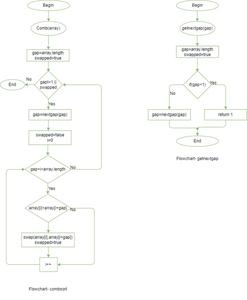Flowchart

Below is the implementation.

## C++

 `// C++ implementation of Comb Sort``#include``using` `namespace` `std;` `// To find gap between elements``int` `getNextGap(``int` `gap)``{``    ``// Shrink gap by Shrink factor``    ``gap = (gap*10)/13;` `    ``if` `(gap < 1)``        ``return` `1;``    ``return` `gap;``}` `// Function to sort a[0..n-1] using Comb Sort``void` `combSort(``int` `a[], ``int` `n)``{``    ``// Initialize gap``    ``int` `gap = n;` `    ``// Initialize swapped as true to make sure that``    ``// loop runs``    ``bool` `swapped = ``true``;` `    ``// Keep running while gap is more than 1 and last``    ``// iteration caused a swap``    ``while` `(gap != 1 || swapped == ``true``)``    ``{``        ``// Find next gap``        ``gap = getNextGap(gap);` `        ``// Initialize swapped as false so that we can``        ``// check if swap happened or not``        ``swapped = ``false``;` `        ``// Compare all elements with current gap``        ``for` `(``int` `i=0; i a[i+gap])``            ``{``                ``swap(a[i], a[i+gap]);``                ``swapped = ``true``;``            ``}``        ``}``    ``}``}` `// Driver program``int` `main()``{``    ``int` `a[] = {8, 4, 1, 56, 3, -44, 23, -6, 28, 0};``    ``int` `n = ``sizeof``(a)/``sizeof``(a);` `    ``combSort(a, n);` `    ``printf``(``"Sorted array: \n"``);``    ``for` `(``int` `i=0; i

## Java

 `// Java program for implementation of Comb Sort``class` `CombSort``{``    ``// To find gap between elements``    ``int` `getNextGap(``int` `gap)``    ``{``        ``// Shrink gap by Shrink factor``        ``gap = (gap*``10``)/``13``;``        ``if` `(gap < ``1``)``            ``return` `1``;``        ``return` `gap;``    ``}` `    ``// Function to sort arr[] using Comb Sort``    ``void` `sort(``int` `arr[])``    ``{``        ``int` `n = arr.length;` `        ``// initialize gap``        ``int` `gap = n;` `        ``// Initialize swapped as true to make sure that``        ``// loop runs``        ``boolean` `swapped = ``true``;` `        ``// Keep running while gap is more than 1 and last``        ``// iteration caused a swap``        ``while` `(gap != ``1` `|| swapped == ``true``)``        ``{``            ``// Find next gap``            ``gap = getNextGap(gap);` `            ``// Initialize swapped as false so that we can``            ``// check if swap happened or not``            ``swapped = ``false``;` `            ``// Compare all elements with current gap``            ``for` `(``int` `i=``0``; i arr[i+gap])``                ``{``                    ``// Swap arr[i] and arr[i+gap]``                    ``int` `temp = arr[i];``                    ``arr[i] = arr[i+gap];``                    ``arr[i+gap] = temp;` `                    ``// Set swapped``                    ``swapped = ``true``;``                ``}``            ``}``        ``}``    ``}` `    ``// Driver method``    ``public` `static` `void` `main(String args[])``    ``{``        ``CombSort ob = ``new` `CombSort();``        ``int` `arr[] = {``8``, ``4``, ``1``, ``56``, ``3``, -``44``, ``23``, -``6``, ``28``, ``0``};``        ``ob.sort(arr);` `        ``System.out.println(``"sorted array"``);``        ``for` `(``int` `i=``0``; i

## Python3

 `# Python program for implementation of CombSort` `# To find next gap from current``def` `getNextGap(gap):` `    ``# Shrink gap by Shrink factor``    ``gap ``=` `(gap ``*` `10``)``/``/``13``    ``if` `gap < ``1``:``        ``return` `1``    ``return` `gap` `# Function to sort arr[] using Comb Sort``def` `combSort(arr):``    ``n ``=` `len``(arr)` `    ``# Initialize gap``    ``gap ``=` `n` `    ``# Initialize swapped as true to make sure that``    ``# loop runs``    ``swapped ``=` `True` `    ``# Keep running while gap is more than 1 and last``    ``# iteration caused a swap``    ``while` `gap !``=``1` `or` `swapped ``=``=` `1``:` `        ``# Find next gap``        ``gap ``=` `getNextGap(gap)` `        ``# Initialize swapped as false so that we can``        ``# check if swap happened or not``        ``swapped ``=` `False` `        ``# Compare all elements with current gap``        ``for` `i ``in` `range``(``0``, n``-``gap):``            ``if` `arr[i] > arr[i ``+` `gap]:``                ``arr[i], arr[i ``+` `gap]``=``arr[i ``+` `gap], arr[i]``                ``swapped ``=` `True`  `# Driver code to test above``arr ``=` `[ ``8``, ``4``, ``1``, ``3``, ``-``44``, ``23``, ``-``6``, ``28``, ``0``]``combSort(arr)` `print` `(``"Sorted array:"``)``for` `i ``in` `range``(``len``(arr)):``    ``print` `(arr[i],end``=``" "``)`  `# This code is contributed by Mohit Kumra`

## C#

 `// C# program for implementation of Comb Sort``using` `System;` `class` `GFG``{``    ``// To find gap between elements``    ``static` `int` `getNextGap(``int` `gap)``    ``{``        ``// Shrink gap by Shrink factor``        ``gap = (gap*10)/13;``        ``if` `(gap < 1)``            ``return` `1;``        ``return` `gap;``    ``}` `    ``// Function to sort arr[] using Comb Sort``    ``static` `void` `sort(``int` `[]arr)``    ``{``        ``int` `n = arr.Length;` `        ``// initialize gap``        ``int` `gap = n;` `        ``// Initialize swapped as true to``        ``// make sure that loop runs``        ``bool` `swapped = ``true``;` `        ``// Keep running while gap is more than``        ``// 1 and last iteration caused a swap``        ``while` `(gap != 1 || swapped == ``true``)``        ``{``            ``// Find next gap``            ``gap = getNextGap(gap);` `            ``// Initialize swapped as false so that we can``            ``// check if swap happened or not``            ``swapped = ``false``;` `            ``// Compare all elements with current gap``            ``for` `(``int` `i=0; i arr[i+gap])``                ``{``                    ``// Swap arr[i] and arr[i+gap]``                    ``int` `temp = arr[i];``                    ``arr[i] = arr[i+gap];``                    ``arr[i+gap] = temp;` `                    ``// Set swapped``                    ``swapped = ``true``;``                ``}``            ``}``        ``}``    ``}` `    ``// Driver method``    ``public` `static` `void` `Main()``    ``{``        ``int` `[]arr = {8, 4, 1, 56, 3, -44, 23, -6, 28, 0};``        ``sort(arr);` `        ``Console.WriteLine(``"sorted array"``);``        ``for` `(``int` `i=0; i

## Javascript

 ``

Output

```Sorted array:
-44 -6 0 1 3 4 8 23 28 56 ```

Illustration:
Let the array elements be

`8, 4, 1, 56, 3, -44, 23, -6, 28, 0`

Initially gap value = 10
After shrinking gap value => 10/1.3 = 7

``` 8 4 1 56 3 -44 23 -6 28 0
-6 4 1 56 3 -44 23  8 28 0
-6 4 0 56 3 -44 23  8 28 1```

New gap value => 7/1.3 = 5;

```-44 4 0 56 3 -6 23 8 28 1
-44 4 0 28 3 -6 23 8 56 1
-44 4 0 28 1 -6 23 8 56 3```

New gap value => 5/1.3 = 3

```-44 1  0 28 4 -6 23 8 56 3
-44 1 -6 28 4  0 23 8 56 3
-44 1 -6 23 4  0 28 8 56 3
-44 1 -6 23 4  0  3 8 56 28```

New gap value => 3/1.3 = 2;

```-44 1 -6 0 4 23 3 8 56 28
-44 1 -6 0 3 23 4 8 56 28
-44 1 -6 0 3 8 4 23 56 28```

New gap value => 2/1.3 = 1;

```-44 -6 1 0 3 8 4 23 56 28
-44 -6 0 1 3 8 4 23 56 28
-44 -6 0 1 3 4 8 23 56 28
-44 -6 0 1 3 4 8 23 28 56

no more swaps required (Array sorted)```

Time Complexity: Average case time complexity of the algorithm is Ω(N2/2p), where p is the number of increments. The worst-case complexity of this algorithm is O(n2) and the Best Case complexity is O(nlogn).
Auxiliary Space : O(1).

## Quiz on Comb Sort

This article is contributed by Rahul Agrawal. If you like GeeksforGeeks and would like to contribute, you can also write an article and mail your article to review-team@geeksforgeeks.org. See your article appearing on the GeeksforGeeks main page and help other Geeks.

Snapshots: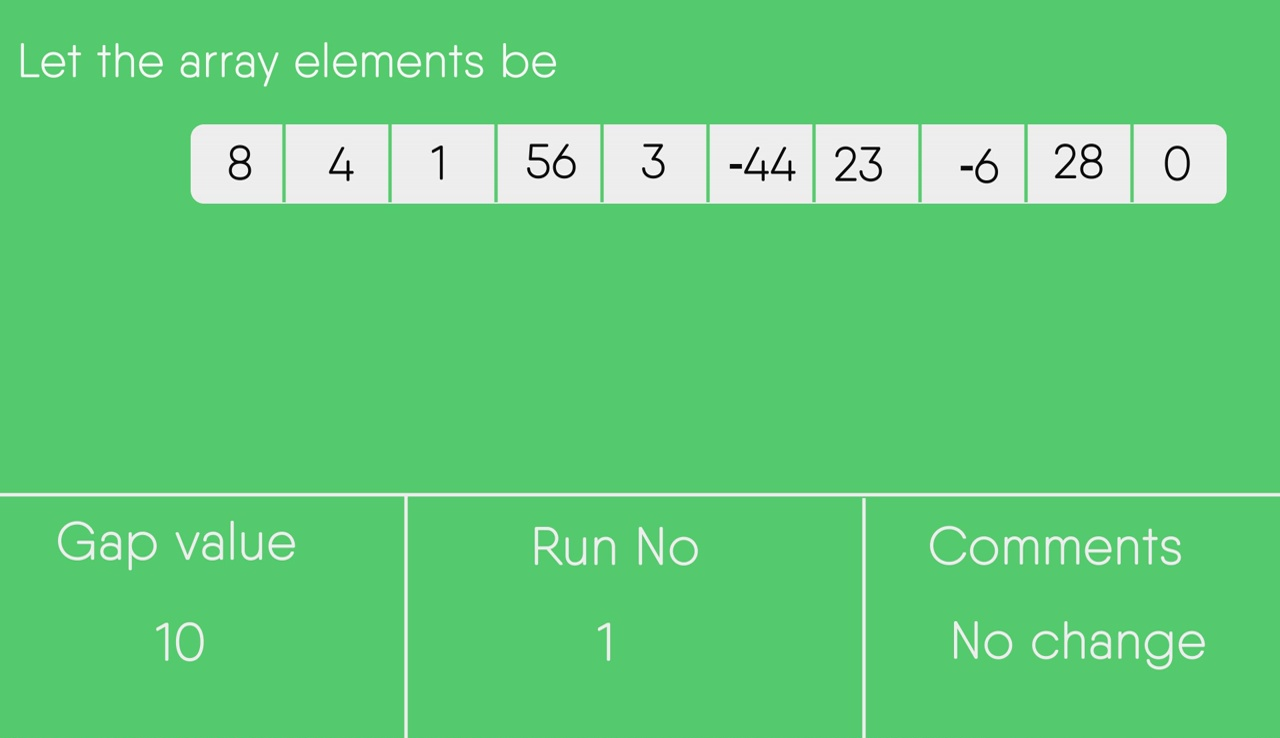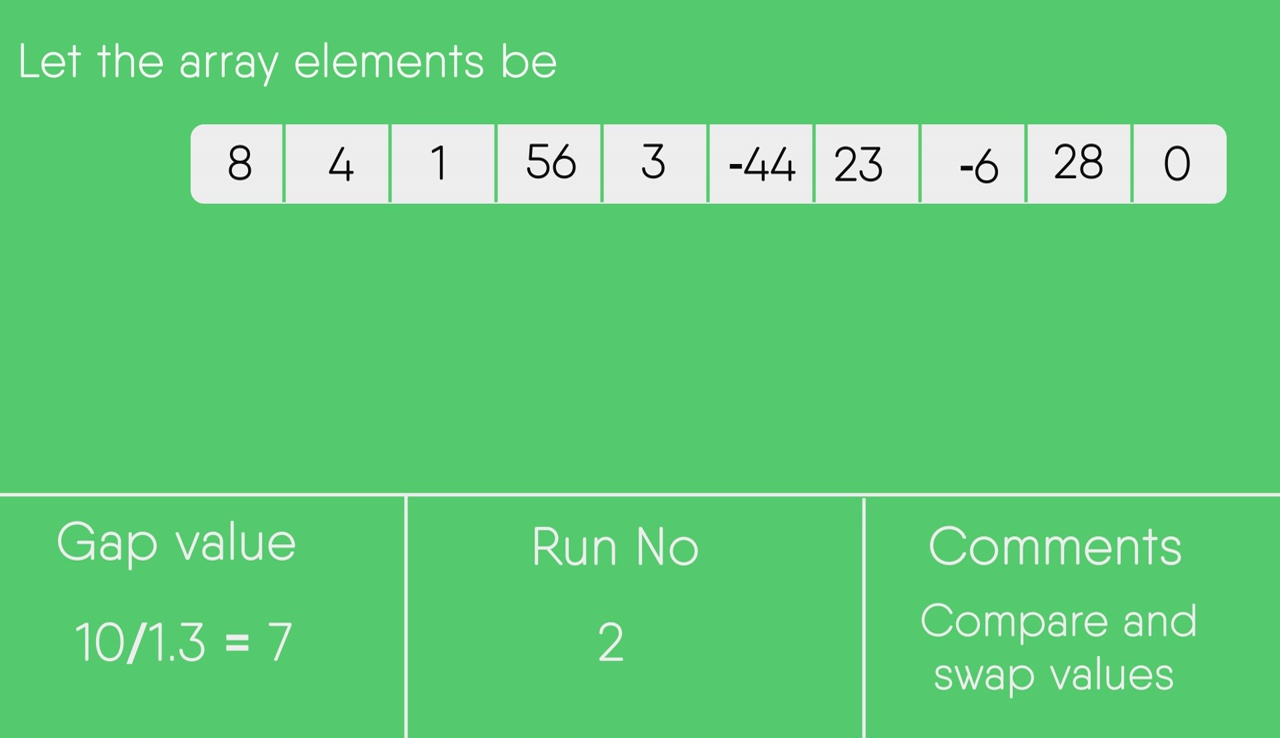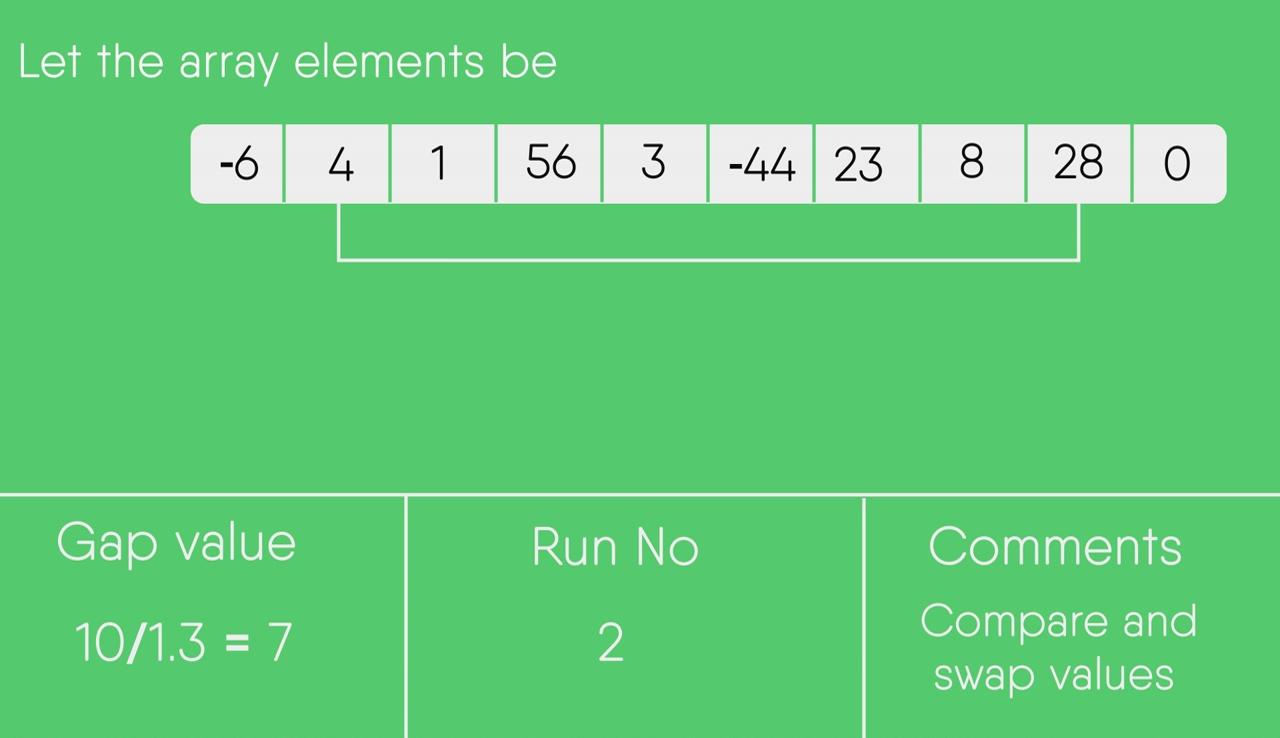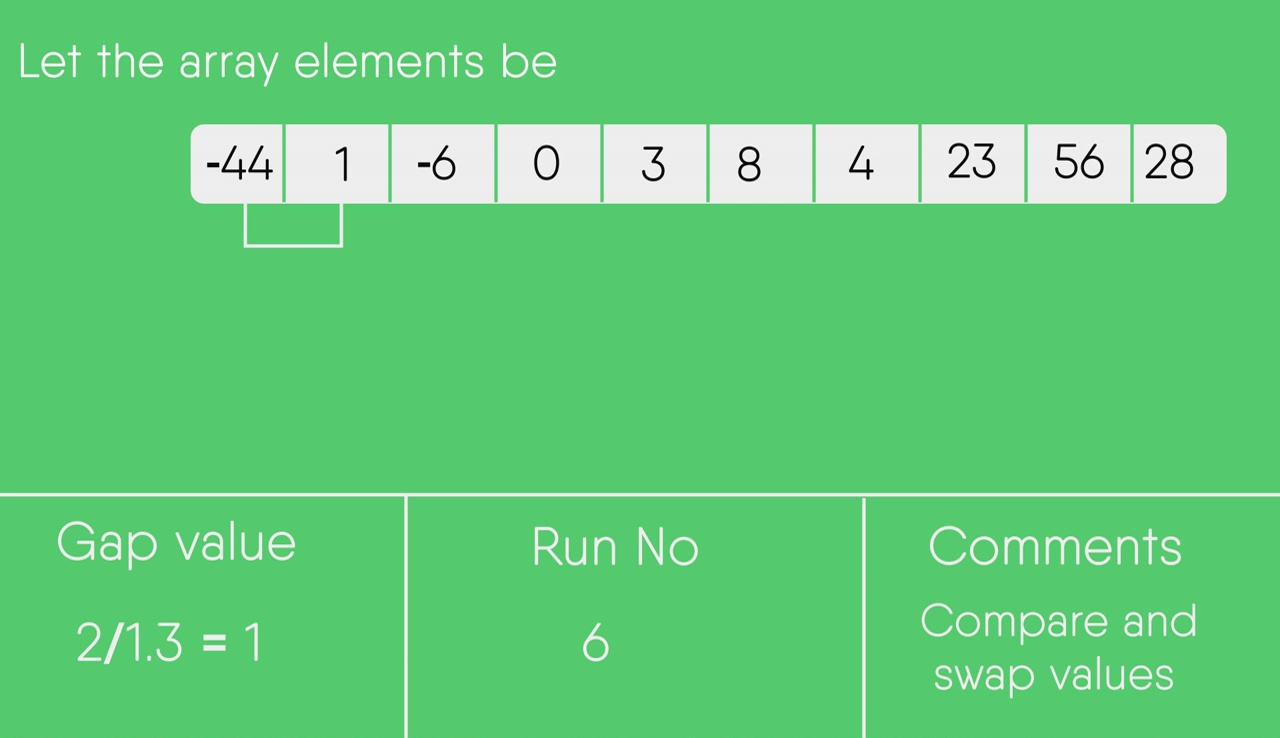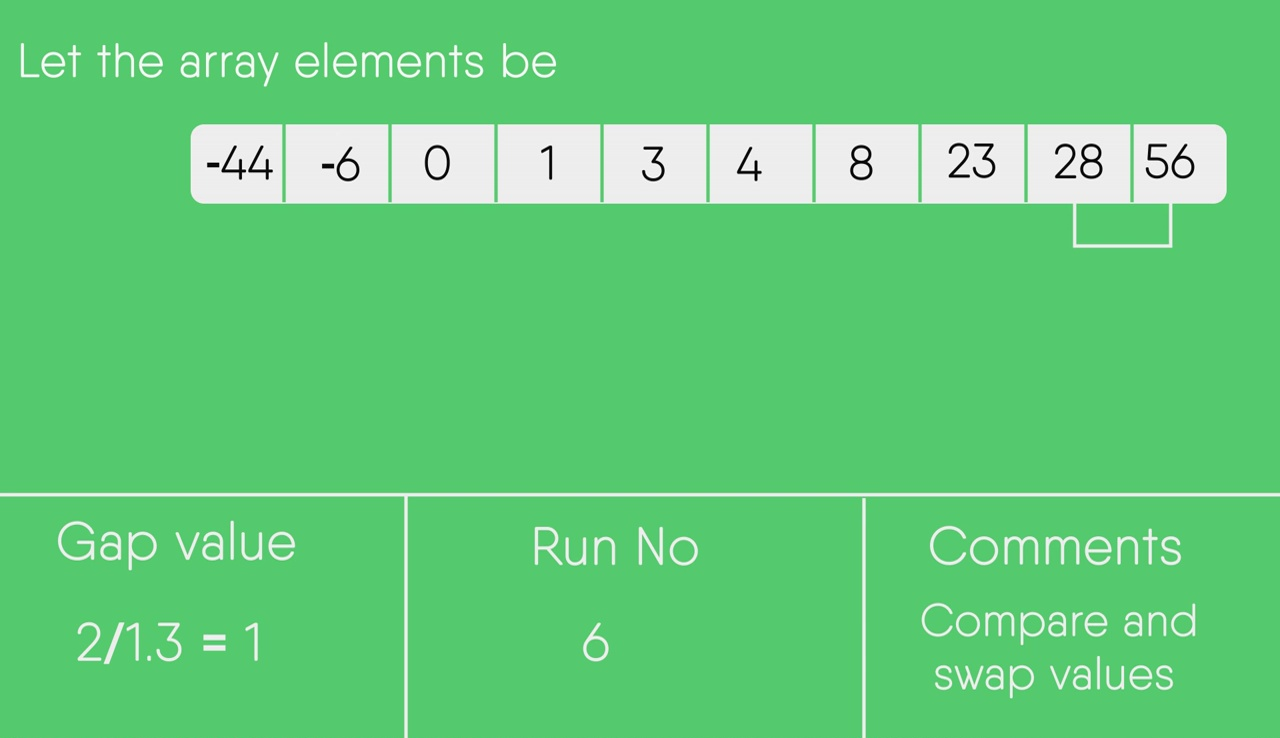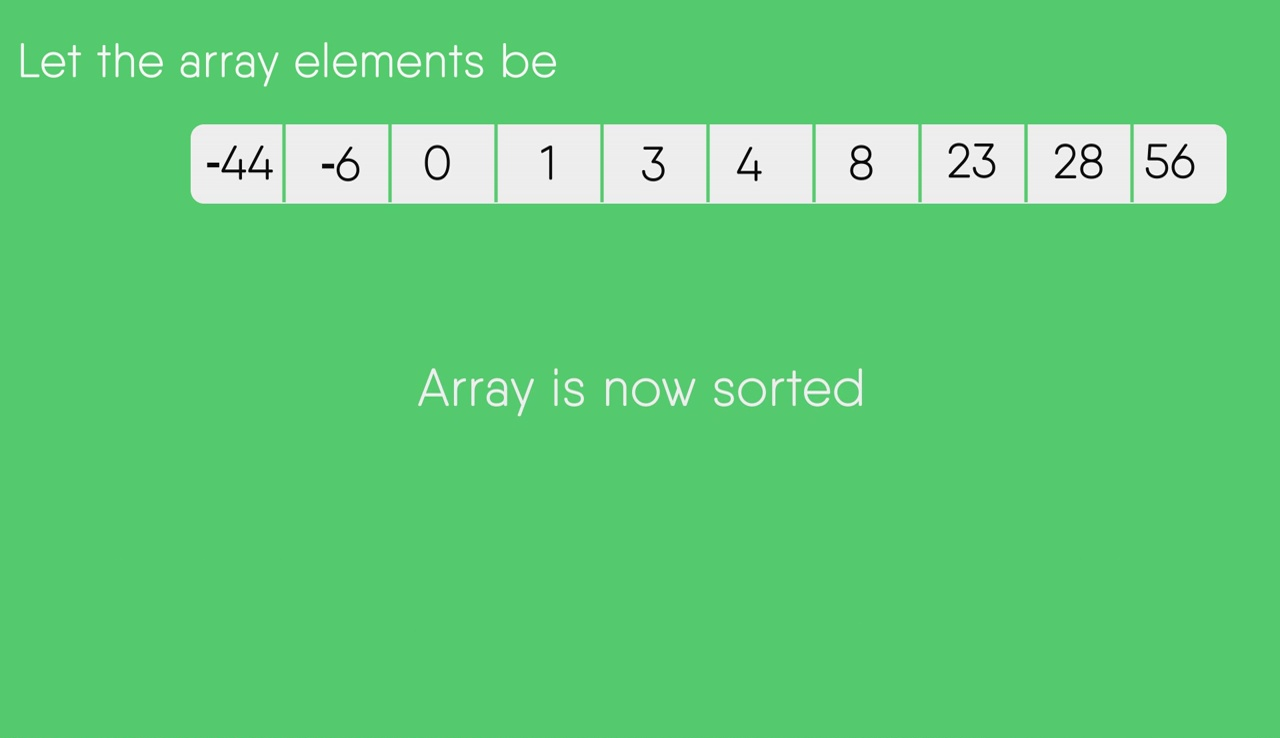### Check out DSA Self Paced Course

Other Sorting Algorithms on GeeksforGeeks/GeeksQuiz
Selection Sort, Bubble Sort, Insertion Sort, Merge Sort, Heap Sort, QuickSort, Radix Sort, Counting Sort, Bucket Sort, ShellSort, Pigeonhole Sort
Please write comments if you find anything incorrect, or you want to share more information about the topic discussed above.

My Personal Notes arrow_drop_up